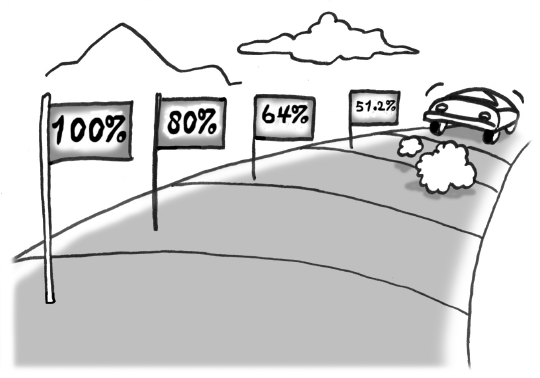### Home > AC > Chapter 13 > Lesson 13.OF2-S > Problem7-138

7-138.

A rule-of-thumb used by car dealers is that the trade-in value of a car decreases by $20\%$ of its value each year.

1. Explain how the phrase “decreases by $20\%$ of its value each year” tells you that the trade-in value varies exponentially with time (i.e., can be represented by an exponential function).

“Decreases by $20\%$” means that you multiply by $0.8$ each year.A sequence with a multiplier is geometric and has an exponential rule.

2.Suppose the initial value of your car is $23,500$. Write an equation expressing the trade-in value of your car as a function of the number of years from now.

$y=23500(0.8)^x$

3. How much will your car be worth in four years?

Use the equation that you found in part (b).

4. In how many years will the trade-in value of your car be $6000$?

$6000=23500(0.8)^x$

Divide by $23,500$ first then take the log of both sides.

5. If your car is really 2.7 years old now, what was its trade-in value when it was new?

This was $2.7$ years ago, so let $x=−2.7$ in the equation.